info@biomedres.us   +1 (720) 414-3554
One Westbrook Corporate Center, Suite 300, Westchester, IL 60154, USA

# Biomedical Journal of Scientific & Technical Research

October, 2021; Volume 39, 2; pp 31200-31208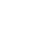Mini Review

Mini Review

# The Quadratic forms of Equations for Calculation of the Ki and Ka Constants of Enzyme Inhibition and Activation

### Krupyanko VI*

Author Affiliations

GK Skryabin Institute of Biochemistry and Physiology of Microorganism, Russian Academy of Sciences, Russia

Received: September 29, 2021 | Published: October 08, 2021

Corresponding author: Krupyanko VI, GK Skryabin Institute of Biochemistry and Physiology of Microorganism, Russian Academy of Sciences, 142290 Pushchino, Moscow region, prospect Nauki 5Postal address: town Pushchino, Prospekt Nauki 5, Moscow region, Russia

DOI: 10.26717/BJSTR.2021.39.006281

#### ABSTRACT

The analysis of dependence of the length projection of Li vectors of biparametrical inhibited and activated (La) enzymatic reactions from the length projection of vectors of monoparametrical inhibited and activated enzymatic reactions on the basic 0 σ plane in three-dimensionalcoordinate system, allows to deduct the quadratic forms of equations for the calculation of the constants of inhibition (Ki) and activation (Ka) of enzymes. Examples of calculation of constants are given.

#### Introduction

In previous articles [1-9], devoted to construction of a vector method representation of enzymatic reactions in the threedimensionalcoordinate system the properties of L vectors of enzymatic reactions was analyzed, from which the parametriacal classification of the types of enzymatic reactions and the equations for calculation of initial activated (Va ) and inhibited (Vi ) reaction rates was suggested. In these article the equations of traditional form (t.f.) for calculation of the constants of activation (Ka) and absent in practice nontrivial types of biparametrical constants of inhibition (Ki) of enzymes (Table 1), was deduced.
This work is devoted to deduction of quadratic form (q.f.) of the equations for calculation of biparametrical constants of inhibition and activation of enzymes (Table 1t & 1f), opening additional ability in the analysis of enzyme action what help of quadratic forms of equation (Table 1q& 1f).
The examples of comparative using traditional and quadratic form of equations for calculation of Ki and Ka constants of inhibition and activation are given.

Table 1: Equations for calculation of Ki and Ka constants (in traditional form).

*The symbol of a graph in Figs. 1-15 corresponds to the type of reaction under study. For example: the line (0) characterizes the position of initial (nonactivated) enzymatic reaction, line I – the position of a graph representing the type of activated enzymatic reaction etc.

#### Deduction of Traditional form of Equations

From (Figures 1, 1a and 2) it easy to see, that ( Ii l ) length of (LIi) projection of LIi vector of biparametrically coordinated, i I type (or mixed type [10 -12]) of enzyme inhibition on Pi semiaxis will be determined by divide (i-0) parameters on ( Ii l ) length of (LIi) projection of LIi vector of – by summation of the quadratic (l2) lengths (orthogonal between them self) LIIIi and LIVi projections of monoparametrical LIIIi and LIVi vectors of i III andtype of enzyme inhibition, (which also are the coordinate of these vectors) but in the same time they taking adjacent place relative to orthogonal LIi projection of LIi vector (Figure 2), determined by equation:

Figure 1: Three dimensional (incompletely) system of rectangular coordinate with separately Pi and Pa semiaxes of molar concentrations of [i] inhibitor and [a] activator. Only 8 L vectors of enzymatic reactions (the symbols: LIi, LIVi, LIIIi, LIIi, LIa, LIIIa, LIIa placed in appropriate parallelepipeds and four orthogonal projections of these L vectors (the symbols: LIi, LIVi, LIIIi and LIa) are placed on basic 0 σ plane (Io, IIo … quadrants of this plane), the magnitude of ϕ angle about 3400.

Figure 1a: Three dimensional (completely)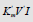coordinate system, (the same as Figure 1), bat with all 14 branched L vectors (7 type of additional L vectors placed without of appropriate arallelepipeds. The ends of all mobile L vectors are joined by dash line (broken part – activated, unbroken part– L vectors of inhibited reactions). The 15th L0 vector of initial reaction (and it L0 projection take place in P point of coordinate intersection. The all 14 orthogonal LIi, LIVi … LIa , LIa projections of L vectors on basic 0 σ plane, are placed completely in (Figure 2).quadrants of transient σ " plane, VIIa/Vi σ and Va/VIIi σ – beginning and finishing ends of the line of orthogonal σ " transient plane projection on basic σ0 plane (in Figures.1a and 2, market by broken lines), the magnitude of ϕ angle about 3400.

Figure 2: Two-dimensional (scalar)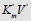coordinate system. The symbols of kinetic parameters: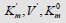the projections LIi, LIVi… LIa, LIVa of three-dimensional vectors: LIi, LIVi… LIa, LIVa on the basic 0 σ plane and symbols of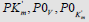coordinate semiaxes the same as in Figure. 1 and in the text, the magnitude of ϕ angle about 150.

The Ii l length of LIi projection on 0 σ plane of Figures.; (1 - 2) may be determined as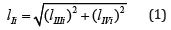Having expressed from Eq. (2) the lIIIi length of LIIIi projection of LIIIi vector on P0V semiaxis ofcoordinate (Figures 1, 1a):from Eq. (3) – the lIVi length of the second adjacent of LIVi vector projection onsemiaxis:and substituted them in Eq. (4):we shall obtain traditional form (t.f.) of equation for calculation of the Ii K constant of biparametrically coordinated, i I type, inhibition of enzymes, taking in to consideration the lIi length of orthogonal projection of LIi vector on basic 0 σ plane of Figure (1a):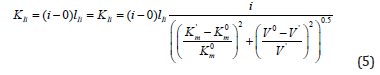where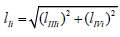, as it follows from (Figures 1&2).

It is analogous for length of adjacent projections: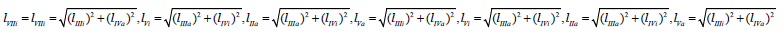for all other lIIa, lVa … of vectors projections of biparametrical reactions (Figures 1,1a & 2).

#### Deduction of Quadratic form of Equations

From analysis of Equations (1-4) one can easily see that substitution in Eq. (4) of the dimensionless coordinates of the lengths of LIIIi and LIVi vector projections is equal to substitution in this equation of the i /KIIIi and i /KIVi parameters then it is not difficult to become the alternative equations for calculation of i K and a K constants of biparametrical types of inhibition and activation of enzymes. Having substituted in Eq. (4) of the dimensionless coordinates of the lengths of LIIIi and LIVi vector projections is equal to substitution in this equation of the i /KIIIi and i /KIVi parameters.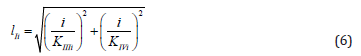we find that such as:this substitution will lead to equation: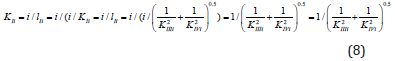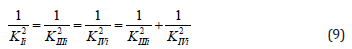convenient for calculation of constant inhibition of enzymes (Eq. 1, q.f., in Table 1).

It is analogous for all the other equations of biparametrical types of inhibition (Eqs: 2, 5 – 7), and activation (Eqs: 9 – 11 and 14-15 of enzymes, Table 1q & 1f) such as orthogonal projections of correspond L vectors on the basic 0 σ plane, easy to determine by data of two-dimensional (scalar)coordinate system (Figure 2), taking into account orthogonal L projections of tree-dimensional L vectors on basic 0 σ plane of (Figures 1a).

Examples of constants calculation.

### Example 1: Calculation of Constant Inhibition

The inhibitory effect of Tungstic acid anions 2 4 4 WO − (0.5×10− M) on the initial rate of pNPP cleavage by calf alkaline phosphatase (Figure 3). shows that the presence 0.5×10−4M of these anions in the enzyme-substrate system makes the binding of the enzyme to the substrate cleaved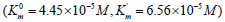difficult and leads to a decrease in the maximum reaction rate (V0= 2.56, V’= 1.74μmol/ (min per μg protein). This meets all the features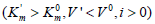of the biparametrically coordinated, i I type, of enzyme inhibition (Table 1, line 1). Hence, to calculate the Ii K constant of this phosphatase inhibition it is necessary to use Eq. (5, text), or (Eq. 1, Table 1t & 1f).

Figure 3: Inhibitory effect of anions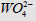on the initial rate V0, mol/ (min per g protein) of pNPP cleavage by calf alkaline phosphatase. Note: line 1 – the concentration ofis 0.5×10−4M ; line (0) – the inhibitor is absent.

Substitution in this equation of the parameters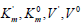and i obtained by data analysis of (Figure 3) allows the calculation of this constant of enzyme inhibition:Substitution the same parameters (recalculated to values of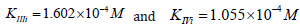constants) in equation (1 of Table 1t & 1f), result into next value of this Ii K constant: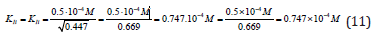Substitution of these parameters in (Eq. 1, q.f., Table 1)result into the same value of the constant of enzyme inhibition: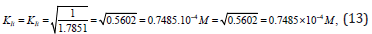From Eqs. (10 -13) it follows that dimension of constants in all cases, are the molar concentration of inhibitor: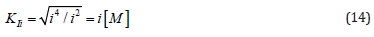Control. Determine the value of the IIIi K constant of this experiment (Figure 3) by values of Ii K and IVi K constants.

From equations (11) and (12), rewritten to the form,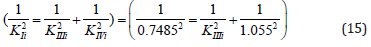it follows that: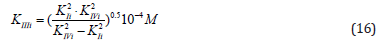Substitution the necessary parameters from (Eq. 15) to (Eq. 16), we find that: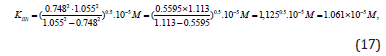which is in good agreement with the experimental value of this constant (Eq. 12).

### Example 2: Calculation of Constant Inhibition

The inhibitory effect of Pyrrolidine dithiocarbonic acid (PDTA) on the initial rate of pNPP cleavage by canine alkaline phosphatase shows that in the presence of 1×10−3M PDTA the parameters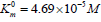and V0= 2.921 μmol/(min per μg protein) change as follows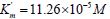and V ' = 3.616 μmol/(min per μg protein) (Figure 4). This corresponds to the, Vi type, of enzyme pseudoinhibition(Table 1, line 5) and Eq. (5, t.f.) is applicable for calculation of the KVi constant of enzyme inhibition. Substitution all necessary parameters in this equation allows calculation of this constant of enzyme inhibition:

Figure 4: Inhibitory effect of PDTA on the initial rate V0 , mol/(min per g protein) of pNPP cleavage by canine alkaline phosphatase. Note: line 1 – the concentration of PDTA is 1×10−3M ; line (0) – the inhibitor is absent.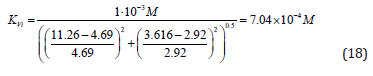Substitution of recalculated parameters of (Figure 4)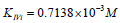and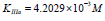to (Eq. 5, Table 1, q.f.) – result into value of Vi K constant inhibition:### Example 3: Calculation of Constant Activation

The activating effect of Guanosine (Guo) on canine alkaline phosphatase (Figure 5) shows that in the presence of 1×10−3M Guo the parameters of initial reaction of pNPP cleavage, i. e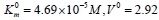μmol/(min per μg protein), change as follows: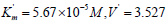μmol/(min per μg protein). This corresponds to the, Ia type, of unassociative enzyme activation.

Figure 5: Activating effect of Guo on the initial rate v0, mol/(min per g protein) of pNPP cleavage by canine alkaline phosphatase. Note: line 1 – the concentration of Guo is 1×10−3M ; line (0) - the activator is absent.

Hence, to calculate the KIIa constant of enzyme activation, one should use Eq. (14, t.f., Table 1).

Substitution of the obtained values of parameters in this equation allows calculation of this constant of enzyme activation: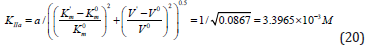Substitution of the:parameters of this experiment (Figure 5) in (Eq. 14, q.f., Table 1), result in to:as it was to be expected, result in to the same value of activation constant: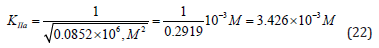From the length parts of equations: (12), (15), (19) and (21) may to see that all they obeys to the signs of Pifagor’s theorem and this may be used as for calculation any of the third constants by the two others known already and for correction the constants, determined by using any other equations.

Example 4: Calculate the value of KIIIi constant of experiment (Figure 3), by value of KIi and KIVi constants. From equation (1, Table 1t & 1f), rewritten to the quadratic form (23).it follows that: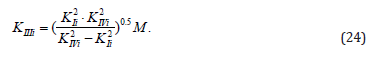Having substitution all necessary parameters from (Eq. 23) to (Eq. 24), we shall become, that: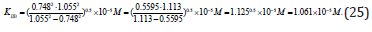Ii is analogous for all biparametrically types of catalyzed reactions (Table 1).

Introduction in practice of quadratic forms of equations for calculation of Ki and Ka constants, will facilitates for many authors to interpret obtained data of nontrivial types of inhibition and activation by such definition as «essentially competitive inhibition», «similarly to competitive inhibition » and so on [13 -17].

Mini Review

# The Quadratic forms of Equations for Calculation of the Ki and Ka Constants of Enzyme Inhibition and Activation

### Krupyanko VI*

Author Affiliations

GK Skryabin Institute of Biochemistry and Physiology of Microorganism, Russian Academy of Sciences, Russia

Received: September 29, 2021 | Published: October 08, 2021

Corresponding author: Krupyanko VI, GK Skryabin Institute of Biochemistry and Physiology of Microorganism, Russian Academy of Sciences, 142290 Pushchino, Moscow region, prospect Nauki 5Postal address: town Pushchino, Prospekt Nauki 5, Moscow region, Russia

DOI: 10.26717/BJSTR.2021.39.006281

#### ABSTRACT

The analysis of dependence of the length projection of Li vectors of biparametrical inhibited and activated (La) enzymatic reactions from the length projection of vectors of monoparametrical inhibited and activated enzymatic reactions on the basic 0 σ plane in three-dimensionalcoordinate system, allows to deduct the quadratic forms of equations for the calculation of the constants of inhibition (Ki) and activation (Ka) of enzymes. Examples of calculation of constants are given.